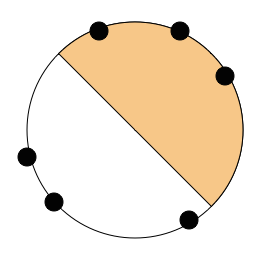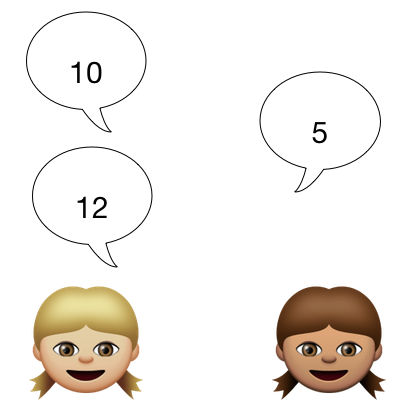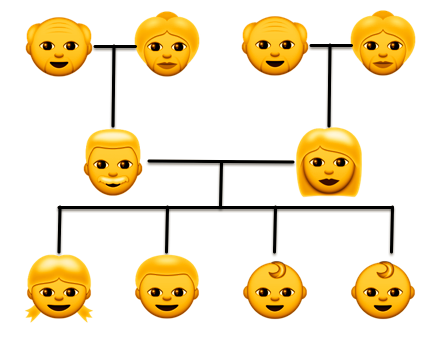Probability

# Pigeonhole Principle Warmup

Danny has a bunch of dice in his drawer. He recalls that 5 of them are green, 6 of them are blue and 7 of them are red. He reaches in and grabs several without looking. How many does he have to grab, in order to ensure that 3 of them are the same color?

5 integers are randomly chosen from 1 to 2015. What is the probability that there is a pair of integers whose difference is a multiple of 4?Find the largest integer $n$ that satisfies the following condition:

If any 6 points are chosen on the perimeter of a circle, then we can draw semicircle of the circle, such that there are at least $n$ points on it.

Note: A point on the perimeter of the semicircle is considered to lie on it.Alice and Betty play a game where Alice goes first. They each say a distinct integer from 1 to 15 (inclusive). The first person to say an integer which, when summed with a previously spoken integer, gives the value of 16, will lose the game.

Who will win the game?What is the minimum number of people that must be in a family, so that it's guaranteed that two of them have the same month of birth?

×